Courses
Courses for Kids
Free study material
Offline Centres
MoreLast updated date: 04th Dec 2023
Total views: 381.9k
Views today: 5.81k

# Find the least number which must be subtracted from each of the following so as to get a perfect square. Also find the square root of the perfect square so obtained.(i) 402(ii) 1989(iii) 3250(iv) 825(v) 400Verified
381.9k+ views
Hint: Use the square root by long division method to find the square roots and the remainder that comes will be the number that should be subtracted to make it perfect square.

First we write the steps of doing long division method for finding square roots:

Step 1: First, group the digits in pairs, starting with the digit in units place. Each pair and the remaining digit (if any) is called a period.

Step 2: Think of the largest number whose square is equal to or just less than the first period. Taking this number as a divisor and also the quotient.

Step 3: Subtract the product of the divisor and the quotient from the first period and bring down the next period to the right of the reminder. This makes a new dividend.

Step 4: Now, the new divisor is obtained by taking two times the quotient and annexing the suitable digit which is also taken as the next digit of quotient, chosen in such a way that the product of new divisor and the digits is equal or just less than the new dividend.

Step 5: Repeat steps (2), (3), (4) till all the periods have been taken up. Now the quotient so obtained is the required square root of the given number.

i) So taking the 1st number 402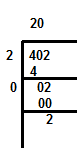So, ‘2’ should be subtracted so that the number becomes a perfect square with square root 20.

ii) Now the 2nd number is 1989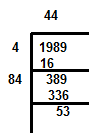So, ‘53’ should be subtracted so that the number becomes perfect with square root 44.

iii) Now the 3rd number is 3250.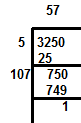So ‘1’ should be subtracted so that the number becomes a perfect square with square root 57.

iv) 4th number is 825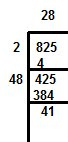So, ‘41’ should be subtracted so that the number becomes a perfect square with square root 28.

v) 5th number is 4000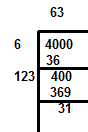So, ‘31’ should be subtracted so that the number becomes a perfect square with square root 63.

Note: Students generally don’t know clearly how to do square root by division method; hence they should follow the steps in the solution so that the concept becomes clear. They should also do calculations clearly to avoid any errors.